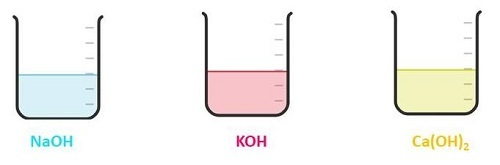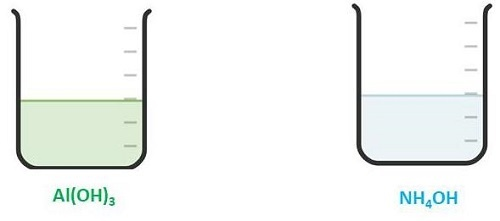# Examples of Bases

## Introduction

Examples of bases with its properties are as follows −

• Bases have pH value more than 7.

• Aqueous solution of basic compounds undergoes ionization and can conduct electricity.

• On reacting base with acids can produce salts.

• The concentrated base or strong base is known as Caustic.

• If base added to alkaline solution it has bitter taste.

• Base get identify easily due to the presence of OH- ions.

• On applying red litmus paper to basic solution it changes the colour into blue.

• On adding to acids compounds base can react vigorously.

• On adding base to water it can conduct electricity.

• It is a slippery substance in nature.

### Classification and Types of Bases

The basic compounds get classified on the basis of its concentration, acidity and ionization degree.

There are three types of bases as per its acidity −

• Monoacidic base – The compounds has only one hydroxide ion (OH-) and able to react with only one hydrogen (H+) ion. Examples: NaOH, KOH, etc.

• Di-acidic base – The compounds has two hydroxide (OH-) ions and able to react with two hydrogen (H+) ions. Examples: $\mathrm{Ca(OH)_2}$.

• Tri-acidic base: The compounds has three hydroxide (OH-) ion and can reacts with three hydrogen (H+) ions. Examples: $\mathrm{Al(OH)_3}$.

Types of bases according to its concentration −

Dilute base: Low concentration of base in solution. Example: Dilute KOH, dilute $\mathrm{Ca(OH)_2}$, etc.

Concentrated base: High concentration of base in solution. Example: Concentrated NaOH, concentrated $\mathrm{Mg(OH)_2}$, etc.

Types of base on it ionization degree −

Strong Base: The base which gets completely ionized in water solution is called strong base. Example: NaOH.

Weak Base: The base which does not completely get ionized into water solution is called weak base. Example: $\mathrm{NH_4OH}$.

## Uses of Bases

• Base like NaOH (sodium hydroxide) is used in preparation of rayon, paper, soap, etc.

• $\mathrm{Mg(OH)_2}$ (magnesium hydroxide) is used as an antacid.

• $\mathrm{Ca(OH)_2}$ (calcium hydroxide) is used as a dry powder mixture for painting and decoration. It is also used in bleaching powder.

• $\mathrm{NH_4OH}$ (ammonium hydroxide) is used in laboratories.

## Examples of Bases

The examples of the various bases are listed below.

### $\mathrm{Ba(OH)_2}$ – Barium Hydroxide

$\mathrm{Ba(OH)_2}$ is a strong base. It is a di-acidic base. It can ionize completely on adding to water and can form OH- ion in water.

### $\mathrm{NH_4OH}$ – Ammonium Hydroxide

$\mathrm{NH_4OH}$ is a weak base. It is a monoacidic base. It cannot completely ionize on adding to water and form very less OH- ions in water solution.

### NaOH – Sodium Hydroxide

NaOH is a strong base. It is a monoacidic base. It get completely dissociate into water. It is also known as caustic soda.

### $\mathrm{Al_2O_3}$ – Aluminium Oxide

$\mathrm{Al_2O_3}$ can act both as acid and base as it is an amphoteric oxide. It can react with strong acid and form salt. Thus it acts like a base.

$$\mathrm{Al_2O_3 + 6 HCl\:\rightarrow\:2 AlCl_3 + 3 H_2O}$$

### CaO – Calcium Oxide

CaO is a strong base. It comes under solid bases. It is a metallic oxide. On reacting CaO with water solution it forms a strong base. It is also known as quicklime.Figure 1: Strong bases with aqueous solution

### $\mathrm{Al(OH)_3}$ – Aluminium Hydroxide

$\mathrm{Al(OH)_3}$ is an amphoteric substance and acts as an acid as well as base. It acts as a weak base. It can react with strong acids.

### KOH – Potassium Hydroxide

KOH is a strong base because it can dissociate totally in water solution. It is monoacidic base and also known as caustic potash.

### $\mathrm{NH_3}$ – Ammonia

Ammonia can act as a lewis base as it can donate its extra electrons to lewis acid and shows basic character.

### $\mathrm{Fe(OH)_3}$ – Iron Hydroxide

$\mathrm{Fe(OH)_3}$ is tri-acidic base. It acts as weak base as it get dissociate incompletely on adding to water solution.

### $\mathrm{Sr(OH)_2}$ – Strontium Hydroxide

The $\mathrm{Sr(OH)_2}$ is a strong base. The hydroxides of group 2nd elements like Sr, Ba and Ca can form strong base.

### $\mathrm{Cu(OH)_2}$ – Copper hydroxide

$\mathrm{Cu(OH)_2}$ is a weak base. It dissociate in very small amount on adding to water. Mostly of part is insoluble.

### BaO – Barium Oxide

BaO is a strong base because it act as a very good proton acceptor.

### $\mathrm{Ca(OH)_2}$ – Calcium Hydroxide

$\mathrm{Ca(OH)_2}$ is a strong di-acidic base. It can completely ionize in water. It is also known as slaked lime.

### $\mathrm{Pb(OH)_2}$ – Lead Hydroxide

$\mathrm{Pb(OH)_2}$ is a weak base as it is rarely soluble in water and form $\mathrm{Pb^{2+}}$ ions in weak acidic solution.

### MgO – Magnesium Oxide

MgO act as an basic oxide as it can produce basic Mg(OH)2 on mixing in water.

### $\mathrm{Mg(OH)_2}$ – Magnesium Hydroxide

$\mathrm{Mg(OH)_2}$ is a strong and di-acidic base. It gets ionized 100% in water solution.

### RbOH – Rubidium Hydroxide

RbOH is a monoacidic base. It is a strong base due to it 100% ionization in water.

### BeO – Berrilium Oxide

BeO is an amphoteric compound. It can act both as an acid and a base.

### $\mathrm{Zn(OH)_2}$ – Zinc Hydroxide

$\mathrm{Zn(OH)_2}$ is a amphoteric substance. It can act both as acid and a base. On reaction with acid it can act as a base.

### NaH – sodium Hydride

NaH is a strong base and dissociate completely on adding to water solution.

### CsOH – Cesium Hydroxide

CsOH is a strong base. It is a monacidic base. Cesium is more electronegative due to which it act as strong base.Figure 2: Weak bases

## Conclusion

Basic compounds are the compounds which can accept protons from other compounds. Bases are differentiate as Lewis base, Arrhenius base or Bronsted-Lowry base. Basic solutions generally show pH value more than 7.

There are various types of bases like monoacidic, di-acidic, tri-acididc, etc. the strong base is that which can completely dissociate in water. The weak bases are those which get incompletely dissociates in water solution. CsOH, NaOH, RbOH, $\mathrm{Mg(OH)_2}$, etc. are the examples of strong bases. $\mathrm{NH_4OH, Cu(OH)_2, Pb(OH)_2}$, etc. are examples of weak bases.

## FAQs

Q1. How could you determine that the compound is basic in nature?

Ans. If the compound shows the ability to accept protons from other compound it shows a basic nature. Also basic compound is determined due to formation of hydroxide ions in aqueous solution.

Q2. What is di-acidic base?

Ans. The compounds which contain two hydroxide ions and can form bonds with two hydrogen ions are called di-acidic base.

Q3. What happen when basic compound added to water?

Ans. When basic compounds get added to water it get dissociates into ions and if OH- ions form it confirms its basic nature.

Q4. CsOH is a strong or weak base?

Ans. CsOH is a strong base because it can completely dissociate in water and form more OH- ions.

Q5. What is lewis base?

Ans. Lewis base is a species which can donates its electrons to a lewis acid.

Updated on: 26-Apr-2023

1K+ Views